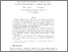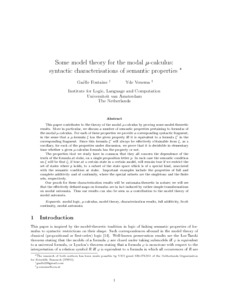# PP-2016-39: Some model theory for the modal mu-calculus: syntactic characterisations of semantic properties

PP-2016-39: Fontaine, Gaëlle and Venema, Yde (2016) Some model theory for the modal mu-calculus: syntactic characterisations of semantic properties. [Pre-print] (Submitted)Preview Text fontaine-venema2.pdf Download (624kB) | Preview

## Abstract

This paper contributes to the theory of the modal $\mu$-calculus by proving some model-theoretic results. More in particular, we discuss a number of semantic properties pertaining to formulas of the modal $\mu$-calculus. For each of these properties we provide a corresponding syntactic fragment, in the sense that a $\mu$-formula $\xi$ has the given property iff it is equivalent to a formula $\xi'$ in the corresponding fragment. Since this formula $\xi'$ will always be effectively obtainable from $\xi$, as a corollary, for each of the properties under discussion, we prove that it is decidable in elementary time whether a given $\mu$-calculus formula has the property or not.

The properties that we study have in common that they all concern the dependence of the truth of the formula at stake, on a single proposition letter $p$. In each case the semantic condition on $\xi$ will be that $\xi$, if true at a certain state in a certain model, will remain true if we restrict the set of states where $p$ holds, to a subset of the state space which is of a special kind, associated with the semantic condition at stake. Important examples include the properties of full and complete additivity and of continuity, where the special subsets are the singletons and the finite sets, respectively.

Our proofs for these characterization results will be automata-theoretic in nature; we will see that the effectively defined maps on formulas are in fact induced by rather simple transformations on modal automata. Thus our results can also be seen as a contribution to the model theory of modal automata.

Item Type: Pre-print PP-2016-39 Prepublication (PP) Series 2016 modal logic, mu-calculus, model theory, characterization results, additivity, Scott continuity, modal automata. ComputationLogic Yde Venema 05 Dec 2016 11:39 05 Dec 2016 13:15 https://eprints.illc.uva.nl/id/eprint/1416View Item